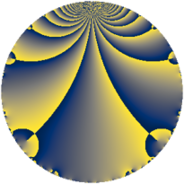# Properties

 Label 6930.2.a.bzLevel $6930$ Weight $2$ Character orbit 6930.a Self dual yes Analytic conductor $55.336$ Analytic rank $0$ Dimension $2$ CM no Inner twists $1$

# Related objects

## Newspace parameters

 Level: $$N$$ $$=$$ $$6930 = 2 \cdot 3^{2} \cdot 5 \cdot 7 \cdot 11$$ Weight: $$k$$ $$=$$ $$2$$ Character orbit: $$[\chi]$$ $$=$$ 6930.a (trivial)

## Newform invariants

 Self dual: yes Analytic conductor: $$55.3363286007$$ Analytic rank: $$0$$ Dimension: $$2$$ Coefficient field: $$\Q(\sqrt{33})$$ Defining polynomial: $$x^{2} - x - 8$$ Coefficient ring: $$\Z[a_1, \ldots, a_{13}]$$ Coefficient ring index: $$2$$ Twist minimal: no (minimal twist has level 2310) Fricke sign: $$-1$$ Sato-Tate group: $\mathrm{SU}(2)$

## $q$-expansion

Coefficients of the $$q$$-expansion are expressed in terms of $$\beta = \sqrt{33}$$. We also show the integral $$q$$-expansion of the trace form.

 $$f(q)$$ $$=$$ $$q + q^{2} + q^{4} - q^{5} + q^{7} + q^{8} +O(q^{10})$$ $$q + q^{2} + q^{4} - q^{5} + q^{7} + q^{8} - q^{10} - q^{11} + ( 1 + \beta ) q^{13} + q^{14} + q^{16} + ( -1 - \beta ) q^{17} + 4 q^{19} - q^{20} - q^{22} + ( 3 + \beta ) q^{23} + q^{25} + ( 1 + \beta ) q^{26} + q^{28} -2 q^{29} + 4 q^{31} + q^{32} + ( -1 - \beta ) q^{34} - q^{35} + ( -1 - \beta ) q^{37} + 4 q^{38} - q^{40} -2 q^{41} - q^{44} + ( 3 + \beta ) q^{46} + q^{49} + q^{50} + ( 1 + \beta ) q^{52} -2 q^{53} + q^{55} + q^{56} -2 q^{58} + 4 q^{59} + 2 q^{61} + 4 q^{62} + q^{64} + ( -1 - \beta ) q^{65} + ( 5 - \beta ) q^{67} + ( -1 - \beta ) q^{68} - q^{70} + 4 q^{71} -2 q^{73} + ( -1 - \beta ) q^{74} + 4 q^{76} - q^{77} - q^{80} -2 q^{82} + 8 q^{83} + ( 1 + \beta ) q^{85} - q^{88} + ( -9 - \beta ) q^{89} + ( 1 + \beta ) q^{91} + ( 3 + \beta ) q^{92} -4 q^{95} + ( 4 - 2 \beta ) q^{97} + q^{98} +O(q^{100})$$ $$\operatorname{Tr}(f)(q)$$ $$=$$ $$2 q + 2 q^{2} + 2 q^{4} - 2 q^{5} + 2 q^{7} + 2 q^{8} + O(q^{10})$$ $$2 q + 2 q^{2} + 2 q^{4} - 2 q^{5} + 2 q^{7} + 2 q^{8} - 2 q^{10} - 2 q^{11} + 2 q^{13} + 2 q^{14} + 2 q^{16} - 2 q^{17} + 8 q^{19} - 2 q^{20} - 2 q^{22} + 6 q^{23} + 2 q^{25} + 2 q^{26} + 2 q^{28} - 4 q^{29} + 8 q^{31} + 2 q^{32} - 2 q^{34} - 2 q^{35} - 2 q^{37} + 8 q^{38} - 2 q^{40} - 4 q^{41} - 2 q^{44} + 6 q^{46} + 2 q^{49} + 2 q^{50} + 2 q^{52} - 4 q^{53} + 2 q^{55} + 2 q^{56} - 4 q^{58} + 8 q^{59} + 4 q^{61} + 8 q^{62} + 2 q^{64} - 2 q^{65} + 10 q^{67} - 2 q^{68} - 2 q^{70} + 8 q^{71} - 4 q^{73} - 2 q^{74} + 8 q^{76} - 2 q^{77} - 2 q^{80} - 4 q^{82} + 16 q^{83} + 2 q^{85} - 2 q^{88} - 18 q^{89} + 2 q^{91} + 6 q^{92} - 8 q^{95} + 8 q^{97} + 2 q^{98} + O(q^{100})$$

## Embeddings

For each embedding $$\iota_m$$ of the coefficient field, the values $$\iota_m(a_n)$$ are shown below.

For more information on an embedded modular form you can click on its label.

Label $$\iota_m(\nu)$$ $$a_{2}$$ $$a_{3}$$ $$a_{4}$$ $$a_{5}$$ $$a_{6}$$ $$a_{7}$$ $$a_{8}$$ $$a_{9}$$ $$a_{10}$$
1.1
 −2.37228 3.37228
1.00000 0 1.00000 −1.00000 0 1.00000 1.00000 0 −1.00000
1.2 1.00000 0 1.00000 −1.00000 0 1.00000 1.00000 0 −1.00000
 $$n$$: e.g. 2-40 or 990-1000 Significant digits: Format: Complex embeddings Normalized embeddings Satake parameters Satake angles

## Atkin-Lehner signs

$$p$$ Sign
$$2$$ $$-1$$
$$3$$ $$-1$$
$$5$$ $$1$$
$$7$$ $$-1$$
$$11$$ $$1$$

## Inner twists

This newform does not admit any (nontrivial) inner twists.

## Twists

By twisting character orbit
Char Parity Ord Mult Type Twist Min Dim
1.a even 1 1 trivial 6930.2.a.bz 2
3.b odd 2 1 2310.2.a.y 2

By twisted newform orbit
Twist Min Dim Char Parity Ord Mult Type
2310.2.a.y 2 3.b odd 2 1
6930.2.a.bz 2 1.a even 1 1 trivial

## Hecke kernels

This newform subspace can be constructed as the intersection of the kernels of the following linear operators acting on $$S_{2}^{\mathrm{new}}(\Gamma_0(6930))$$:

 $$T_{13}^{2} - 2 T_{13} - 32$$ $$T_{17}^{2} + 2 T_{17} - 32$$ $$T_{19} - 4$$ $$T_{23}^{2} - 6 T_{23} - 24$$ $$T_{29} + 2$$ $$T_{31} - 4$$

## Hecke characteristic polynomials

$p$ $F_p(T)$
$2$ $$( -1 + T )^{2}$$
$3$ $$T^{2}$$
$5$ $$( 1 + T )^{2}$$
$7$ $$( -1 + T )^{2}$$
$11$ $$( 1 + T )^{2}$$
$13$ $$-32 - 2 T + T^{2}$$
$17$ $$-32 + 2 T + T^{2}$$
$19$ $$( -4 + T )^{2}$$
$23$ $$-24 - 6 T + T^{2}$$
$29$ $$( 2 + T )^{2}$$
$31$ $$( -4 + T )^{2}$$
$37$ $$-32 + 2 T + T^{2}$$
$41$ $$( 2 + T )^{2}$$
$43$ $$T^{2}$$
$47$ $$T^{2}$$
$53$ $$( 2 + T )^{2}$$
$59$ $$( -4 + T )^{2}$$
$61$ $$( -2 + T )^{2}$$
$67$ $$-8 - 10 T + T^{2}$$
$71$ $$( -4 + T )^{2}$$
$73$ $$( 2 + T )^{2}$$
$79$ $$T^{2}$$
$83$ $$( -8 + T )^{2}$$
$89$ $$48 + 18 T + T^{2}$$
$97$ $$-116 - 8 T + T^{2}$$